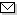Language:   Search:   ContactZentralblatt MATH has released its new interface!
For an improved author identification, see the new author database of ZBMATH.

# Serials and Journals - Browse

Serial or Journal Name starts with:
? A B C D E F G H I J K L M N O P Q R S T U V W X Y Z
Kant-Studien → COMPLETE → ZMATH
Karachi J. Math. → COMPLETE → ZMATH
Karachi Univ. J. Sci. → COMPLETE → ZMATH
Karadeniz Univ. Math. J. → COMPLETE → ZMATH
Kazan. Gos. Univ., Uch. Zap. → COMPLETE → ZMATH
Kazan Tracts Math. → COMPLETE → ZMATH
Kazanĭ, Bull. Soc. phys.-math. (2) → COMPLETE → ZMATH
Keio COE Lect. Ser. Math. Sci. → COMPLETE → ZMATH
Keio Math. Semin. Rep. → COMPLETE → ZMATH
Keio Sci. Technol. Rep. → COMPLETE → ZMATH
Kendall's Libr. Stat. → COMPLETE → ZMATH
Kexue Tongbao, Sci. Bull. → COMPLETE → ZMATH
KIAS Newsl. → COMPLETE → ZMATH
Kibernetika → COMPLETE → ZMATH
Kibern. Sb., Nov. Ser. → COMPLETE → ZMATH
Kibern. Sist. Anal. → COMPLETE → ZMATH
Kibern. Vychisl. Tekh. → COMPLETE → ZMATH
Kibern. Vychisl. Tekh., Kiev → COMPLETE → ZMATH
Kibern. Vychisl. Tekh., Mosk. → COMPLETE → ZMATH
Kinam → COMPLETE → ZMATH
Kinet. Relat. Models → COMPLETE → ZMATH
Kinki Univ. Ser. Quantum Comput. → COMPLETE → ZMATH
Klass. Teor. Veroyatn. Stat. → COMPLETE → ZMATH
Kluwer Int. Ser. Adv. Database Syst. → COMPLETE → ZMATH
Kluwer Int. Ser. Asian Stud. Comput. Inf. Sci. → COMPLETE → ZMATH
Kluwer Int. Ser. Discrete Event Dyn. Syst. → COMPLETE → ZMATH
Kluwer Int. Ser. Eng. Comput. Sci. → COMPLETE → ZMATH
Kluwer Int. Ser. Inf. Retr. → COMPLETE → ZMATH
Kluwer Int. Ser. Softw. Eng. → COMPLETE → ZMATH
Kluwer Int. Ser. Video Comput. → COMPLETE → ZMATH
Kluwer Texts Math. Sci. → COMPLETE → ZMATH
K-Monogr. Math. → COMPLETE → ZMATH
Knižnice Odb. Věd. Spis\uu\ Vys. Uč. Tech., Brno, A → COMPLETE → ZMATH
Knižnice Odb. Věd. Spis\uu\ Vys. Uč. Tech., Brno, B → COMPLETE → ZMATH
Knjižnica Sigma → COMPLETE → ZMATH
Knowl.based Syst. Ser. → COMPLETE → ZMATH
Kobe J. Math. → COMPLETE → ZMATH
Kochi J. Math. → COMPLETE → ZMATH
Kodai Math. J. → COMPLETE → ZMATH
Kōdai Math. Semin. Rep. → COMPLETE → ZMATH
KoG → COMPLETE → ZMATH
Komb. Anal. → COMPLETE → ZMATH
Kompleksn. Sist. Upr. → COMPLETE → ZMATH
Konstr. Teor. Funkts. Funkts. Anal. → COMPLETE → ZMATH
Konuralp J. Math. → COMPLETE → ZMATH
Korean J. Appl. Stat. → COMPLETE → ZMATH
Korean J. Comput. Appl. Math. → COMPLETE → ZMATH
Kragujevac J. Math. → COMPLETE → ZMATH
Kraj. Zadachi Dyfer. Rivnyan' → COMPLETE → ZMATH
Kristallografiya → COMPLETE → ZMATH
\$K\$-Theory → COMPLETE → ZMATH
Kumamoto J. Math. → COMPLETE → ZMATH
Kumamoto J. Sci., Math. → COMPLETE → ZMATH
Kuwait J. Sci. → COMPLETE → ZMATH
Kuwait J. Sci. Eng. → COMPLETE → ZMATH
Kybernetes → COMPLETE → ZMATH
Kybernetik → COMPLETE → ZMATH
Kybernetika → COMPLETE → ZMATH
Kyoto J. Math. → COMPLETE → ZMATH
Kyoyo Ronshu → COMPLETE → ZMATH
Kyungpook Math. J. → COMPLETE → ZMATH
Kyushu J. Math. → COMPLETE → ZMATH
Login Username: Password:

NewsAbel prize 2010I. M. Gelfand 1913-2009MSC2010

HighlightsScientific prize winners of the ICM 2010OverhangLie groups, physics and geometry. An introduction for physicists, engineers and chemists.

Mirror Server

### Mathematical Institute Belgrade [Serbia]

Other Mirror Sites

Copyright © 2019 Zentralblatt MATH | European Mathematical Society | FIZ Karlsruhe | Heidelberg Academy of Sciences
Published by Springer-Verlag | Webmaster